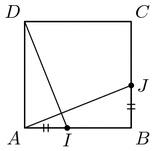Fichier exo_p_scal.mp (figure 3) — Modifié le 20 Juin 2008 à 22 h 21Source

%% MACROS
%% define draw_mark (crossmarks on lines) and similar angle marking operations
%%
%% These macros are stolen from mpman.ps and manfig.mp
marksize:=4pt; % how big the tics on lines are
% how big the arc in an angle is. We define it using := rather than = so that
% it can easily be overridden later if desired.

% don't use this; it is used by draw_marked. Use that instead.
def draw_mark(expr p, a) =
begingroup
save t, dm; pair dm;
t = arctime a of p;
dm = marksize*unitvector direction t of p
rotated 90;
draw (-.5dm.. .5dm) shifted point t of p;
endgroup
enddef;

% draws n tics in the middle of path p
def draw_marked(expr p, n) =
begingroup
save amid;
amid = .5*arclength p;
for i=-(n-1)/2 upto (n-1)/2:
draw_mark(p, amid+.6marksize*i);
endfor
draw p;
endgroup
enddef;

% draw an angle arc and label it with n tics.
def mark_angle(expr a, b, c, n) =
begingroup
save s, p; path p;
p = unitvector(a-b){(a-b)rotated 90}..unitvector(c-b);
s = .9marksize/length(point 1 of p - point 0 of p);
draw_marked(p scaled s shifted b, n);
endgroup
enddef;

% written by DJCM mar 2003
% just like mark_angle but in addition to the n tics also add the
%   label "texts" which is typically           something like    btex $\alpha$ etex
%   at a sensible location near the arc.
default_label_distance_factor := 2.3 ;
label_distance_factor := default_label_distance_factor ;
def label_angle(expr a, b, c, n, texts ) =
begingroup
save s, p; path p;
p = unitvector(a-b){(a-b)rotated 90}..unitvector(c-b);
% the clever notation (x){y}..(z) forces the curve x..z to have tangent y at x.
% (x)..(z) would make the simplest curve from x to z, namely a straight line.
s = .9marksize/length(point 1 of p - point 0 of p);
draw_marked(p scaled s shifted b, n);

save lc ; pair lc ; % label coordinates added by DJCM
ldfs := label_distance_factor*s ;
lc = point 0.5 of ( p scaled ldfs shifted b ) ;
label ( texts , lc );

endgroup
enddef;

def mark_rt_angle(expr a, b, c) =
draw ((1,0)--(1,1)--(0,1))
% not sure what zscaled means
enddef;
%%%%%%%%%%%%%%%%%%%%%%%%%%%%%%%%%%%%%%%%%%%%%%%%%%%%%%%%%%%%%%%%%%%
%%%%%%%%%%%%%%%%%%%%%%%%%%%%%%%%%%%%%%%%%%%%%%%%%%%%%%%%%%%%%%%%%%%%%%%%%%%%%%%%%%%%%%%%%%%%%%%%%%%%%%%%%%%%%%%%%%%%%%%%%%%%%%%%%%%%%%%%%%%%%%%%%%%%%%%%%%%%%%%%%%%%%%%%%%%%%%%%%%%%%%%%%%%%%%%%%%%%%%%%%%%%%%%%%%%%%%%%%%%%%%%%%%%%%%%%%%%%%%%%%%%%%%%%%%%%%%%%%%%%%%%%%%%%

beginfig(1);
u:=1.6cm;
pair A[];
A:=(-1, -1)*u; %A
A:=( 1, -1)*u; %B
A:=( 1, 1)*u;  %C
A:=( -1, 1)*u; %D
draw A--A--A--A--cycle withpen pencircle scaled 0.8bp;;
draw A--A;
%%
label.llft(btex $A$ etex, A);
label.lrt(btex $B$ etex, A);
label.urt(btex $C$ etex, A);
label.ulft(btex $D$ etex, A);
a=0.25;
A:=( -a, a)*u; %M
A:=( -1, a)*u; %Q
A:=( -a, -1)*u; %P
draw A--A;
draw A--A;
draw A--A;
A=whatever[A,A];
A=whatever[A,A];
draw A--A;
mark_rt_angle(A,A,A); %MPD droit
mark_rt_angle(A,A,A); %MQD droit

label.top(btex $\ \,M$ etex, A+(0,0.06u));
label.lft(btex $Q$ etex, A);
label.bot(btex $P$ etex, A);

endfig;

beginfig(2);
u:=3cm;
pair A[];
A:=(0, 0)*u; %O
A:=( 1, 0)*u; %A
A:=( 0, 1)*u; %B
%%%%%%%%%%%%%%%%%%%%%
A:=( 0, 0.1)*u; %
A:=( 0.1, 0)*u; %
A:=( 0.1, 0.1)*u; %

path p;
%p:=A--A--A--A--A--A--A--cycle;
p:=A--A--A--cycle;
draw p rotated -20 withpen pencircle scaled 0.7bp;
draw p rotated 115 scaled 0.7  withpen pencircle scaled 0.7bp;
A:=0.5[Arotated -20,Arotated 115 scaled 0.7];
draw_marked(A--Arotated -20, 1);
draw_marked(A--Arotated -20, 1);
draw_marked(A--Arotated 115 scaled 0.7, 2);
draw_marked(A--Arotated 115 scaled 0.7, 2);
draw Arotated -20--Arotated 115 scaled 0.7;
draw Arotated -20--Arotated 115 scaled 0.7;
draw Arotated -20--Arotated 115 scaled 0.7 dashed evenly scaled 0.7;
draw Arotated -20--Arotated 115 scaled 0.7 dashed evenly scaled 0.7;
mark_rt_angle(A1rotated -20,A0,A2rotated -20);
mark_rt_angle(A1rotated 115,A0,A2rotated 115);
label.rt(btex $A$ etex, Arotated -20);
label.top(btex $B$ etex, Arotated -20);
label.top(btex $C$ etex, Arotated 115 scaled 0.7);
label.bot(btex $D$ etex, Arotated 115 scaled 0.7);
label.bot(btex $O$ etex, A);
dotlabel.top(btex $I$ etex, A);

endfig;

beginfig(3);
u:=1cm;
pair A[];
A:=(-1, -1)*u; %A
A:=( 1, -1)*u; %B
A:=( 1, 1)*u;  %C
A:=( -1, 1)*u; %D
A:=A0+0.4*(A-A);% mil AB
A:=A1+0.4*(A-A);% mil BC

draw A--A--A--A--cycle withpen pencircle scaled 0.7bp;
draw A--A; draw A--A;
draw_marked(A0--A4,2);
draw_marked(A1--A5,2);
%%
label.llft(btex $A$ etex, A);
label.lrt(btex $B$ etex, A);
label.urt(btex $C$ etex, A);
label.ulft(btex $D$ etex, A);
dotlabel.rt(btex $J$ etex, A);
dotlabel.bot(btex $I$ etex, A);

endfig;

beginfig(4);
u:=1.5cm;
pair A[];
A:=(0, 0)*u; %A
A:=( 1.5, 0)*u; %B
A:=( 2, 1)*u;  %C
A:=( 0.5, 1)*u; %D
draw A--A--A--A--cycle withpen pencircle scaled 0.7bp;
label.bot(btex $A$ etex, A);
label.lrt(btex $B$ etex, A);
label.urt(btex $C$ etex, A);
label.top(btex $D$ etex, A);
endfig;
end